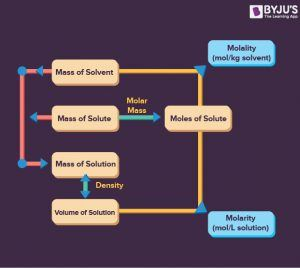# Molality

## What is Molality?

Molality is defined as the “total moles of a solute contained in a kilogram of a solvent.”

Molality is also known as molal concentration. It is a measure of solute concentration in a solution. The solution is composed of two components; solute and solvent. There are many different ways to express the concentration of solutions like molarity, molality, normality, formality, volume percentage, weight percentage and part per million. The term needs to calculate the mass of the solvent and moles of solute.

### Molality Formula

$$\begin{array}{l} Molality \left ( M \right ) = \frac {Number \ of \ moles \ of \ solute}{Mass \ of \ solvent \ in \ kgs}\end{array}$$

$$\begin{array}{l} Molality \left ( M \right ) = \frac{g \times 1000}{W \times m}\end{array}$$

## Recommended Videos## Difference Between Molarity and Molality

 Molality Molarity Mass of the solvent Volume of the whole solution Unit sign expressed as $$\begin{array}{l}\small \left (m \right )\end{array}$$ Unit sign expressed as $$\begin{array}{l}\small \left ( M \right )\end{array}$$ It has units of $$\begin{array}{l}\small moles / kg\end{array}$$ It has units of $$\begin{array}{l}\small moles / liter \end{array}$$Molality

## Molality Examples

Question: Calculate the molality of a solution where 0.5 grams of toluene (C7H8) is dissolved in 225 grams of Benzene (C6H6). Calculate the moles of given solute.

Solution:

Toluene: Molecular weight =

$$\begin{array}{l}\small C_{7}H_{8} = 7 \times 12 + 1 \times 8 = 92 \ grams/mole\end{array}$$

Using the formula:

$$\begin{array}{l}\small Moles \ of \ Toluene = \frac{Mass\ in\ grams}{Molecular\ weight} = \frac{5.0\ grams}{92\ grams/mole} = 0.054 \ mole.\end{array}$$

So, the mole of toluene is

$$\begin{array}{l}\small 0.054 \ mole.\end{array}$$

Now calculate the kilogram of solvent.

$$\begin{array}{l}\small \frac{225\ grams\ of Benzene}{1000} = 0.225 \ kilogram\end{array}$$

As the final step, calculate the molality using the formula.

$$\begin{array}{l}\small Molality \left (m \right ) = \frac{Moles\ of\ Toluene}{Mass\ of\ Benzene\ in\ grams}\end{array}$$

$$\begin{array}{l}\small Molality \left (m \right ) = \frac{0.054 \ moles}{0.225 \ kg}\end{array}$$
$$\begin{array}{l}\small Molality \left (m \right ) = 0.24 \ m\end{array}$$

Q1

### What are Molality and Molarity?

Molarity is the number of moles of a substance per litre of solution, also known as molar concentration. A capital M signifies solutions labelled with molar concentration. A 1.0 M solution contains 1 mole of solvent per litre of solution. Molality is the number of solvent moles per kilogram.

Q2

### What Does Molality mean?

Molality is a solution property and is defined as the number of solvent moles per kilogram. Molality’s SI unit is mol/kg. A solution with a 3 molar/kg molality is often defined as “3 molal” or “3 m.” However, it is now preferred following the unit SI system, mol/kg or a similar SI unit.

Q3

### What is Molality used for?

Molality is the preferred concentration transmission approach because the solution’s mass of solute and solvent does not change.

Q4

### What is the advantage of Molarity over Molality?

Practically testing in a laboratory is better. Even though due to temperature differences affecting volume in molarity and not molality, it is not as reliable as molality. Molarity error sources from volume estimation are also greater than errors from a molality balance.

Q5

### How is molarity useful?

Molarity (M) is one of the most common units used to quantify the concentration of a solution, representing the number of moles of solute per litre of solution (moles / Litre).